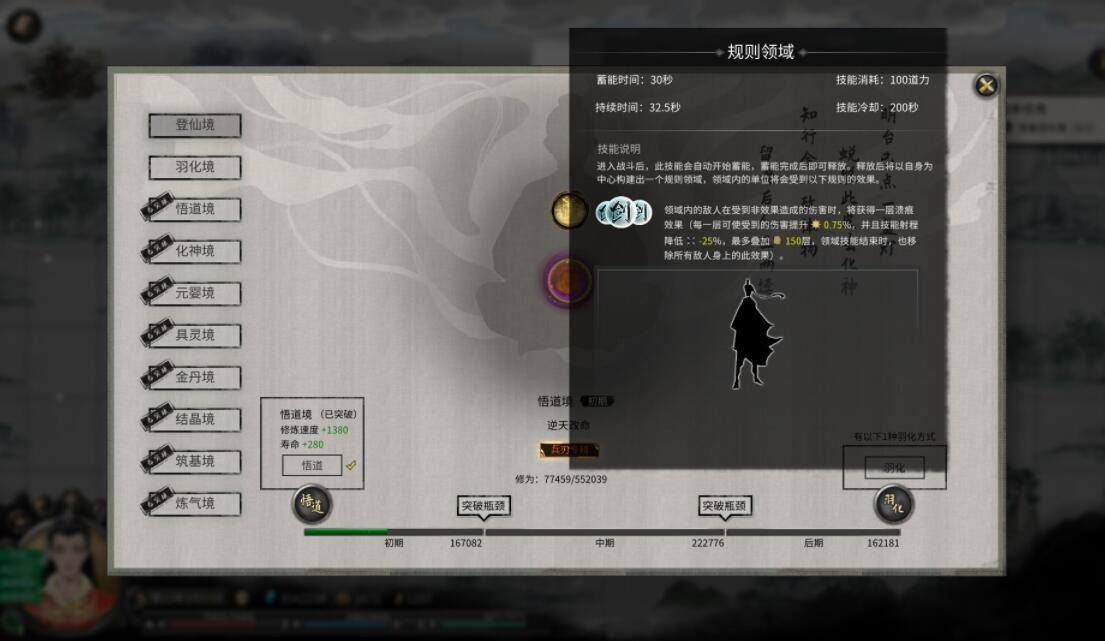• 注册
• # 鬼谷八荒道力值怎么恢复

• 查看作者
• 打赏作者
• 当前位置： 省街博客 > 知识大全 > 正文

在鬼谷八荒游戏中道力值的恢复是非常有讲究的，甚至它还有着专门的计算公式，而玩家只需要根据专有的数据算法，就能够精准地计算出自己所需要的道力值恢复数据啦！## 鬼谷八荒道力值恢复计算方法介绍：

一个月回30，查看方法是在自己人物属性那里，血蓝下面多了个道力，每当你跳月就会回30，一定要省着点，别乱用，领域是关键战斗救命用的。### 道力值上限计算公式

道魂里面不含有剑或雷属性时

把1属性道魂记作1，把2属性道魂记作2，把3属性道魂记作3。

测试数据如下：

1+2+3=180

1+1+2=150

1+1+3=150

2+2+1=150

2+2+3=210

3+3+1=210

3+3+2=240

推算出掌、指、枪、拳、刀、火、水、土、木、风属性，加30道力值(基础自带的30道力值)。

若计算总值小于150道力值时，则向上取值记为150，如，1+1+1=90<150，1+1+2=120<150，均向上取值记为150。

若计算总值大于或等于150道力值时，取值记为计算总值，如，1+2+3=180，取值记为180。

道魂里面含有剑或雷属性时

测试数据如下：

剑水+剑水+剑=210

剑水+剑+剑=180

剑+剑+剑=150

雷水+雷水+雷=210

雷水+雷+雷=180

雷+雷+雷=150

推算出剑、雷属性，加50道力值(基础自带的30道力值+特殊加成的20道力值)，如：

剑水+剑水+剑=50+30+50+30+50=210

剑水+剑+剑=50+30+50+50=180

剑+剑+剑=50+50+50=150

雷水+雷水+雷=50+30+50+30+50=210

雷水+雷+雷=50+30+50+50=180

雷+雷+雷=50+50+50=150

游戏的玩法多种多样，不少玩家刚加入都会遇到大大小小的难题。各位萌新玩家们，省街博客拥有这款游戏的详细攻略，会持续帮助你解决这些难题。

请登录之后再进行评论

• 偏好设置
• 做任务
• 到底部
• 帖子间隔 侧栏位置：